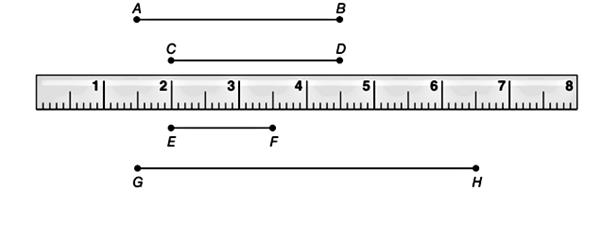Chapter 1.2, Problem 17E### Elementary Geometry for College St...

6th Edition
Daniel C. Alexander + 1 other
ISBN: 9781285195698

#### Solutions

Chapter
Section### Elementary Geometry for College St...

6th Edition
Daniel C. Alexander + 1 other
ISBN: 9781285195698
Textbook Problem
1 views

# Judging from the ruler shown (not to scale), estimate the measure of each line segment. a) A B b) C DTo determine

a) To estimate:

The measure of the line segment AB.

Explanation

Calculation:

Given,

Here, the point A of the line segment is at 1.5 cm and the point B is at 4.5 cm, thus the length of AB¯ is given by

AB=4

To determine

b) To estimate:

The measure of the line segment CD.

### Still sussing out bartleby?

Check out a sample textbook solution.

See a sample solution

#### The Solution to Your Study Problems

Bartleby provides explanations to thousands of textbook problems written by our experts, many with advanced degrees!

Get Started

#### Find more solutions based on key concepts

Elementary Technical Mathematics

#### CHECKPOINT 3. If the demand functions for two products are

Mathematical Applications for the Management, Life, and Social Sciences

#### In Exercises 1520, simplify the expression. 18. (2x3) (3x2) (16x1/2)

Applied Calculus for the Managerial, Life, and Social Sciences: A Brief Approach

#### The slope of the tangent line to r = cos θ at is:

Study Guide for Stewart's Multivariable Calculus, 8th